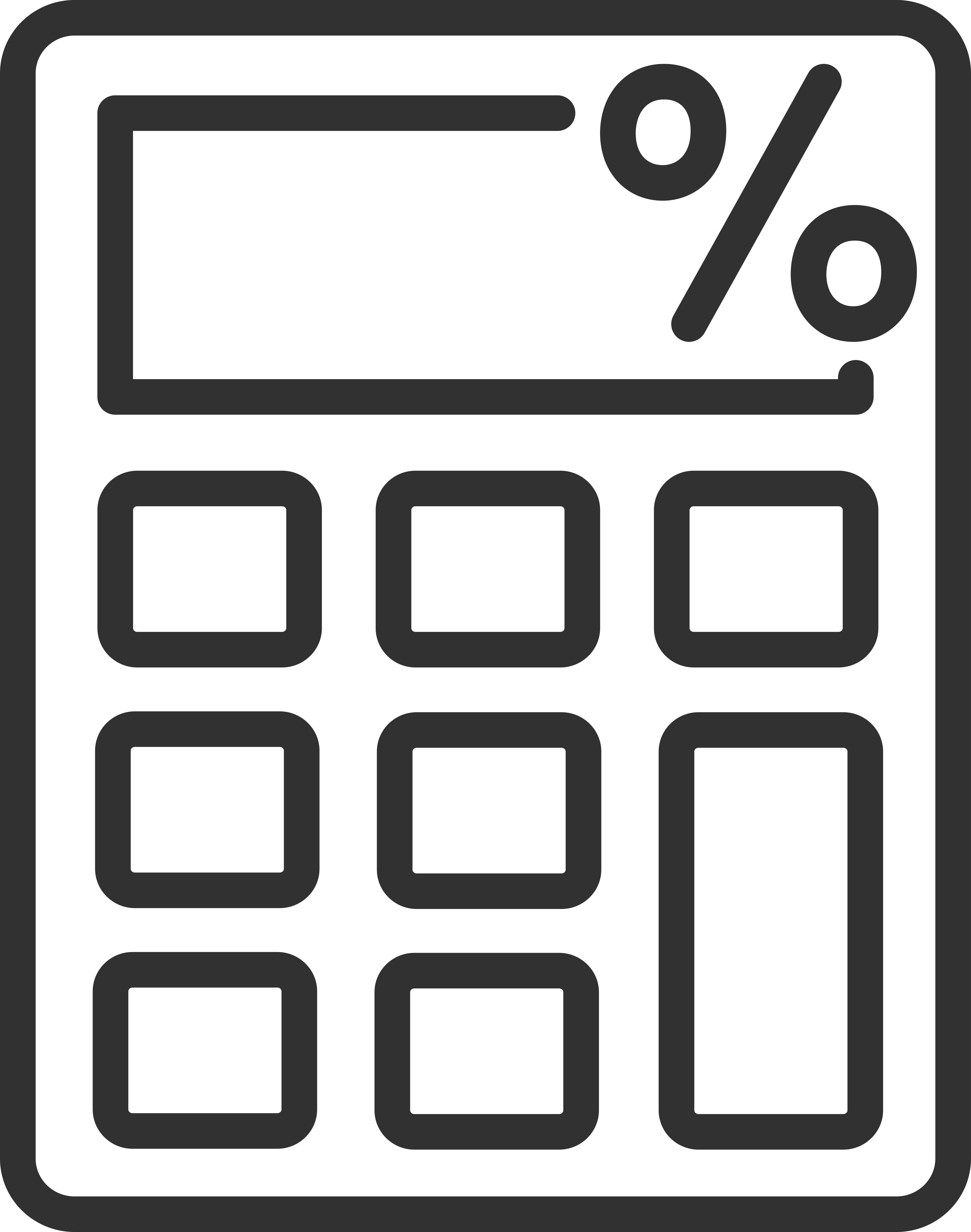Percentage Calculators

Our percentage calculators and resources are useful when working with percentages or converting values to and from a percentage. From finding the percentage of a value or converting a fraction or decimal to a percentage there’s a tool for you.

Use our other math calculators to solve more math problems.